# To draw: The graph for the function f ( x ) = x − 2 ln x .### Single Variable Calculus: Concepts...

4th Edition
James Stewart
Publisher: Cengage Learning
ISBN: 9781337687805### Single Variable Calculus: Concepts...

4th Edition
James Stewart
Publisher: Cengage Learning
ISBN: 9781337687805

#### Solutions

Chapter 5.1, Problem 6E

(a)

To determine

## To draw:The graph for the function f(x)=x−2lnx.

Expert Solution

The graph for the function f(x)=x2lnx is drawn.

### Explanation of Solution

Given information:

The curve function is f(x)=x2lnx.

The region lies in the interval 1x5. So the limits are a=1 and b=5.

Draw the graph for the function f(x)=x2lnx lies the interval 1x5 as shown in Figure 1.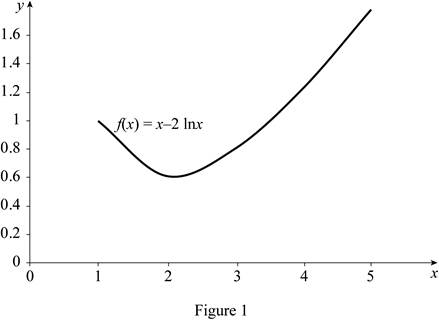(b)

(i)

To determine

### The area under the graph of f using right endpoints and four rectangles.

Expert Solution

The area under the graph of f using right endpoints and four rectangles is 4.44.

### Explanation of Solution

Draw the graph for the function f(x)=x2lnx with four rectangles using the right endpoints as shown in Figure 2.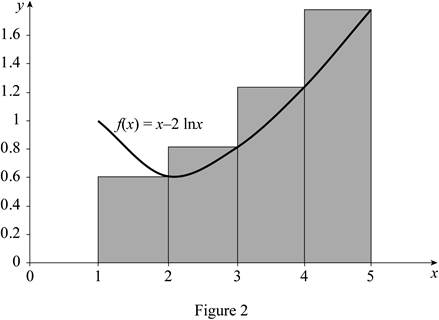The expression to find the estimate of the areas of n rectangles (Rn) using right endpoints is shown below:

Rn=f(x1)Δx+f(x2)Δx+...+f(xn)Δx (1)

Here, the right endpoint height of the first rectangle is f(x1), the width is Δx, the right endpoint height of the second rectangle is f(x2), and the right endpoint height of the nth rectangle is f(xn).

Find the width (Δx) using the relation:

Δx=ban (2)

Here, the upper limit is b, the lower limit is a, and the number of rectangles is n.

Find the area estimate for four rectangles with right end points:

Substitute 5 for b, 1 for a, and 4 for n in Equation (2).

Δx=514=1

Refer to Figure 2.

Take the right endpoint height of the first rectangle’s f(x1) value as 0.6, the right endpoint height of the second rectangle’s f(x2) value as 0.82, the right endpoint height of the third rectangle’s f(x3) value as 1.22, and the right endpoint height of the fourth rectangle’s f(x4) value as 1.8.

Substitute 4 for n, 0.6 for f(x1), 1 for Δx, 0.82 for f(x2), 1.22 for f(x3), and 1.8 for f(x4) in Equation (1).

R4=(0.6×1)+(0.82×1)+(1.22×1)+(1.8×1)=0.6+0.82+1.22+1.8=4.44

Therefore, the area under the graph of f using right endpoints for n=4 is 4.44.

(ii)

To determine

### The area under the graph of f using midpoints and four rectangles.

Expert Solution

The area under the graph of f using midpoints and four rectangles is 3.93.

### Explanation of Solution

Draw the graph for the function f(x)=x2lnx with four rectangles using midpoints as shown in Figure 3.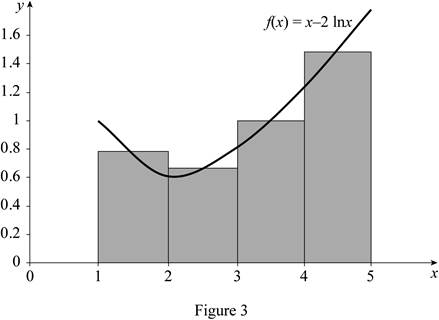The expression to find the estimate of the areas of n rectangles (Mn) using midpoints is shown below:

Mn=f(x1)Δx+f(x2)Δx+...+f(xn)Δx (3)

Here, the midpoint height of the first rectangle is f(x1), the width is Δx, the midpoint height of the second rectangle is f(x2), and the midpoint height of nth rectangle is f(xn).

Find the area estimate for four rectangles with mid points:

Refer to Figure 3.

Take the midpoint height of the first rectangle’s f(x1) value as 0.78, the midpoint height of the second rectangle’s f(x2) value as 0.67, the midpoint height of the third rectangle’s f(x3) value as 1, and the midpoint height of the fourth rectangle’s f(x4) value as 1.48.

Substitute 4 for n, 0.78 for f(x1), 1 for Δx, 0.67 for f(x2), 1 for f(x3), and 1.48 for f(x4) in Equation (3).

M4=(0.78×1)+(0.67×1)+(1×1)+(1.48×1)=0.78+0.67+1+1.48=3.93

Therefore, the area under the graph of f using mid points for n=4 is 3.93.

(c)

(i)

To determine

### The area under the graph of f using right endpoints and eight rectangles.

Expert Solution

The area under the graph of f using right endpoints and eight rectangles is 3.94.

### Explanation of Solution

Draw the graph for the function f(x)=x2lnx with eight rectangles using the right endpoints as shown in Figure 4.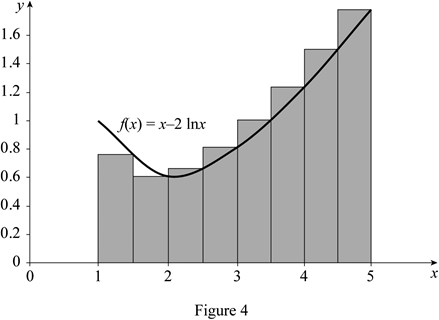Find the area estimate for eight rectangles with right end points:

Substitute 5 for b, 1 for a, and 8 for n in Equation (2).

Δx=518=0.5

Refer to Figure 4.

Take the right endpoint height of the first rectangle’s f(x1) value as 0.76, the right endpoint height of the second rectangle’s f(x2) value as 0.6, the right endpoint height of the third rectangle’s f(x3) value as 0.68, the right endpoint height of the fourth rectangle’s f(x4) value as 0.82, the right endpoint height of the fifth rectangle’s f(x5) value as 1, the right endpoint height of the sixth rectangle’s f(x6) value as 1.22, the right endpoint height of the seventh rectangle’s f(x7) value as 1.5, and the right endpoint height of the eighth rectangle’s f(x8) value as 1.8.

Substitute 8 for n, 0.76 for f(x1), 0.5 for Δx, 0.6 for f(x2), 0.68 for f(x3), 0.82 for f(x4), 1 for f(x5), 1.22 for f(x6), 1.5 for f(x7), and 1.8 for f(x8) in Equation (1).

R8=[(0.76×0.5)+(0.6×0.5)+(0.68×0.5)+(0.82×0.5)+(1×0.5)+(1.22×0.5)+(1.5×0.5)+(1.8×0.5)]=0.38+0.3+0.34+0.41+0.25+0.61+0.75+0.9=3.94

Therefore, the area under the graph of f using right endpoints for n=8 is 3.94.

(ii)

To determine

### The area under the graph of f using midpoints and eight rectangles.

Expert Solution

The area under the graph of f using midpoints and eight rectangles is 3.975.

### Explanation of Solution

Draw the graph for the function f(x)=x2lnx with eight rectangles using midpoints as shown in Figure 5.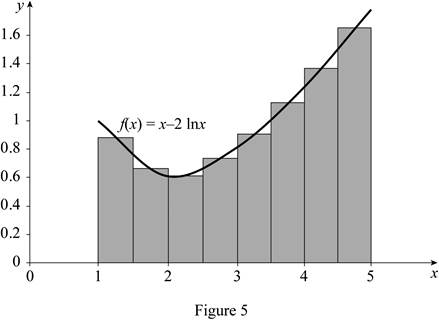Find the area estimate for eight rectangles with mid points:

Refer to Figure 5.

Take the midpoint height of the first rectangle’s f(x1) value as 0.87, the midpoint height of the second rectangle’s f(x2) value as 0.68, the midpoint height of the third rectangle’s f(x3) value as 0.6, the midpoint height of the fourth rectangle’s f(x4) value as 0.74, the midpoint height of the fifth rectangle’s f(x5) value as 0.9, the midpoint height of the sixth rectangle’s f(x6) value as 1.12, the midpoint height of the seventh rectangle’s f(x7) value as 1.38, and the midpoint height of the eighth rectangle’s f(x8) value as 1.66.

Substitute 8 for n, 0.87 for f(x1), 0.5 for Δx, 0.68 for f(x2), 0.6 for f(x3), 0.74 for f(x4), 0.9 for f(x5), 1.12 for f(x6), 1.38 for f(x7), and 1.66 for f(x8) in Equation (3).

M8=[(0.87×0.5)+(0.68×0.5)+(0.6×0.5)+(0.74×0.5)+(0.9×0.5)+(1.12×0.5)+(1.38×0.5)+(1.66×0.5)]=0.435+0.34+0.3+0.37+0.45+0.56+0.69+0.83=3.975

Therefore, the area under the graph of f using mid points for n=8 is 3.975.

### Have a homework question?

Subscribe to bartleby learn! Ask subject matter experts 30 homework questions each month. Plus, you’ll have access to millions of step-by-step textbook answers!